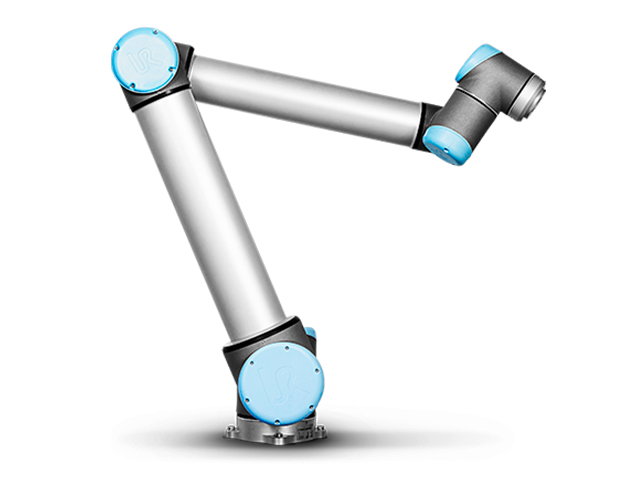$$\newcommand{\dint}{\mathrm{d}} \newcommand{\vphi}{\boldsymbol{\phi}} \newcommand{\vpi}{\boldsymbol{\pi}} \newcommand{\vpsi}{\boldsymbol{\psi}} \newcommand{\vomg}{\boldsymbol{\omega}} \newcommand{\vsigma}{\boldsymbol{\sigma}} \newcommand{\vzeta}{\boldsymbol{\zeta}} \renewcommand{\vx}{\mathbf{x}} \renewcommand{\vy}{\mathbf{y}} \renewcommand{\vz}{\mathbf{z}} \renewcommand{\vh}{\mathbf{h}} \renewcommand{\b}{\mathbf} \renewcommand{\vec}{\mathrm{vec}} \newcommand{\vecemph}{\mathrm{vec}} \newcommand{\mvn}{\mathcal{MN}} \newcommand{\G}{\mathcal{G}} \newcommand{\M}{\mathcal{M}} \newcommand{\N}{\mathcal{N}} \newcommand{\S}{\mathcal{S}} \newcommand{\diag}{\mathrm{diag}(#1)} \newcommand{\diagemph}{\mathrm{diag}(#1)} \newcommand{\tr}{\text{tr}(#1)} \renewcommand{\C}{\mathbb{C}} \renewcommand{\R}{\mathbb{R}} \renewcommand{\E}{\mathbb{E}} \newcommand{\D}{\mathcal{D}} \newcommand{\inner}{\langle #1 \rangle} \newcommand{\innerbig}{\left \langle #1 \right \rangle} \newcommand{\abs}{\lvert #1 \rvert} \newcommand{\norm}{\lVert #1 \rVert} \newcommand{\two}{\mathrm{II}} \newcommand{\GL}{\mathrm{GL}} \newcommand{\Id}{\mathrm{Id}} \newcommand{\grad}{\mathrm{grad} \, #1} \newcommand{\gradat}{\mathrm{grad} \, #1 \, \vert_{#2}} \newcommand{\Hess}{\mathrm{Hess} \, #1} \newcommand{\T}{\text{T}} \newcommand{\dim}{\mathrm{dim} \, #1} \newcommand{\partder}{\frac{\partial #1}{\partial #2}} \newcommand{\rank}{\mathrm{rank} \, #1}$$

# UR 10 Robot

## Introduction

The main objective is to define the inverse kinematic model of the UR 10 robot.This robot is mainly used for pick and place applications in industry.

## Direct Geometric Model

In order to define the geometric model of robot in the space, we need to associate for each joint of the robot a frame. For this we will use the Denavit-Hartenberg parameters. In the following video, this parametrization is explained on a simple robot:

## Inverse Kinematic Model

In order to calculate the Inverse Kinematic model of a 6 axis robot, we need to "inverse" the direct geometric model of the robot. In the following video, a methodology is proposed: During the process, we need to solve some trigonometric equations. In the following video, some explanations are given so as to solve these equations:

## Exercice[]

•             
import numpy as np
from math import factorial as fac

def binomial(n,i):
try:
binom=fac(n)/(fac(i)*fac(n-i))
except ValueError:
binom=0.0
return binom

def bernstein(n,i,t):
#TO DO
return





•             
import numpy as np

def decast(t,P):
#TO DO
return




•             
import numpy as np
import matplotlib.pyplot as plt

def DrawBezier(P,nb=100):
#TO DO
return





## References

1. J.H. Ahlberg, E.N. Nilson & J.L. Walsh (1967) The Theory of Splines and Their Applications. Academic Press, New York.
2. C. de Boor (1978) A Practical Guide to Splines. Springer-Verlag.
3. G.D. Knott (2000) Interpolating Cubic Splines. Birkhäuser.
4. H.J. Nussbaumer (1981) Fast Fourier Transform and Convolution Algorithms. Springer-Verlag.
5. H. Späth (1995) One Dimensional Spline Interpolation. AK Peters.